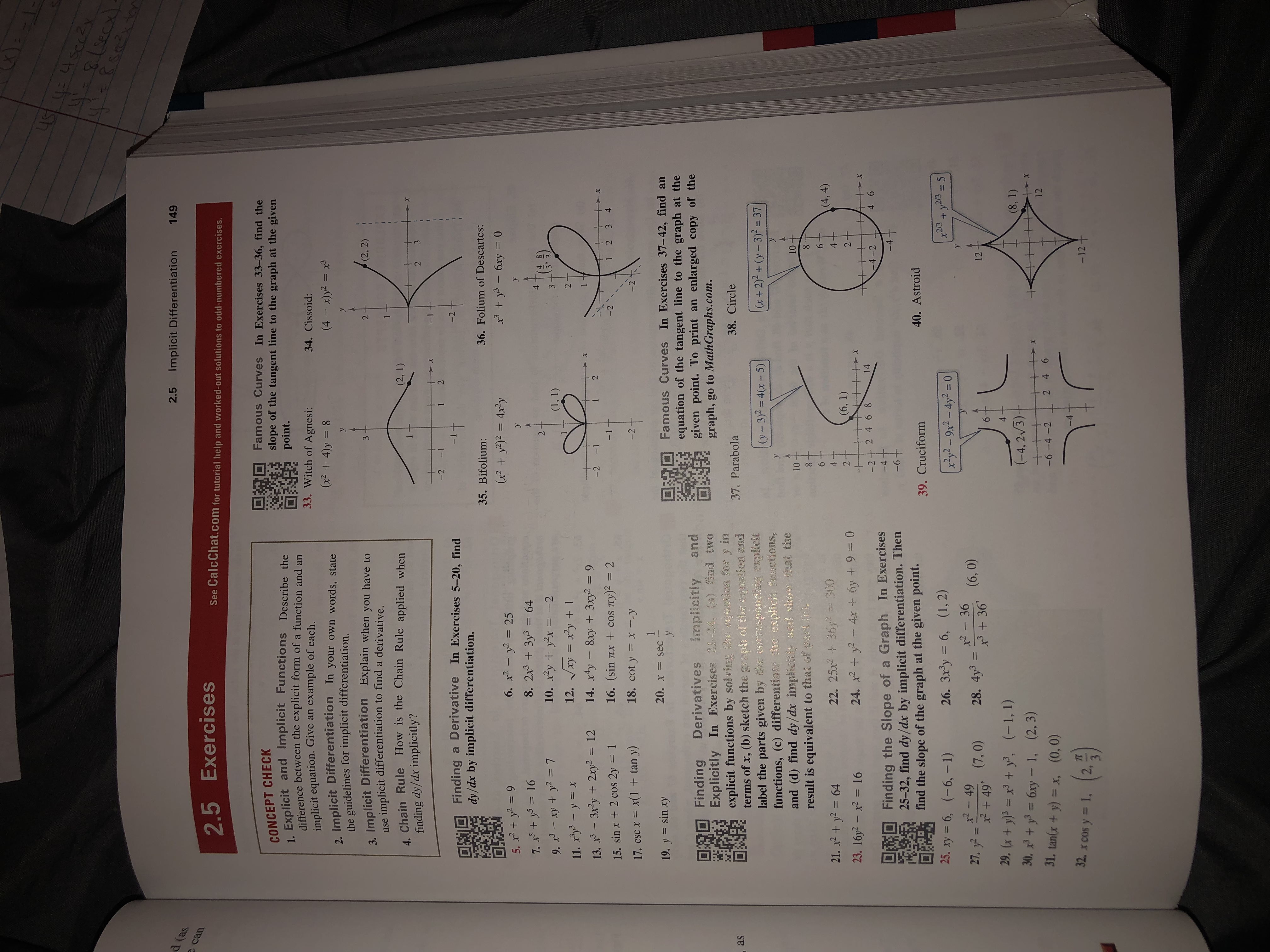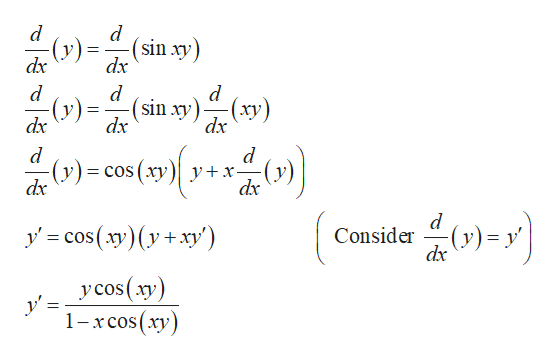# uSsecs.aixtoఇనడెర2.5Implicit Differentiation149d (ascan2.5 ExercisesSee CalcChat.com for tutorial help and worked-out solutions to odd-numbered exercises.Famous Curves In Exercises 33-36, find theslope of the tangent line to the graph at the givenpoint.CONCEPT CHECK1. Explicit and Implicit Functions Describe thedifference between the explicit form of a function and an33. Witch of Agnesi:34. Cissoid:implicit equation. Give an example of each.(x2 4)y = 8(4 x)y2 = x32. Implicit Differentiationthe guidelines for implicit differentiation.3 Implicit Differentiation Explain when you have toimplicit differentiation to find a derivative.1In your own words, stateyy(2, 2)23useChain Rule How is the Chain Rule applied when(2, 1)finding dy/dx implicitly?322-2 -1Derivative In Exercises 5-20, find-2Findingdy/dx by implicit differentiation.36. Folium of Descartes:35. Bifolium:3y36xy0(x y2) = 4xy6. x2-y2255. x+297. xy169. x3-xyy211y8. 2x3 + 3y3= 64410. xyy2x -212. Vxy = y + 173(1, 1)211. xy-y x14. xy 8xy+3xy2 =9Ty) 213. x312y2xy2 122- 1-2X16. (sin4321TIX +COS15. sinx +2 cos 2y18. cot y-2x --y17. CSC x x(1 + tan y)120. x sec -yFamous Curves In Exercises 37-42, find anequation of the tangent line to the graph at thegiven point. To print angraph, go to Math Graphs.com.19. y sinxyandenlarged copy of theFinding DerivativesExplicitly In Exercises 31-24, (a) find twoexplicit functions by solving he cquation for y interms of x, (b) sketch the graps of the cquatken andlabel the parts given by orraspondisg srxplicitfunctions, (c) differentia dhe explicit fn ctions,and (d) find dy/dx implicisy and show ihat theresult is equivalent to that of park (e).Implicitly38. Circle37. Parabolaas(x+2)2+(y-3)2= 37(y-3)2= 4(x-5)1010(4, 4)22. 25x2 y 30021. x2+ y26423. 16y2- 2 = 16(6, 1)4x + 6y +9= 024. xy2+14-2+ 2 46 84 6-4-2w1Finding the Slope of a Graph In Exercises25-32, find dy/dx by implicit differentiation. Thenfind the slope of the graph at the given point.-4-6t40. Astroid39. Cruciformx2/3y2/3 525. xy = 6, (-6, - 1)26. 3x3y = 6, (1, 2)x2y2-9x2-4y2 - 0x2-36x2-4927. y2x49' (7,0)29. (x + y)3 = x3 + y, (-1, 1)30. y36xy - 1, (2, 3)28. 4y3111236 (6,0)4(8, 1)(-4, 2/3)+2 46Xx1231. tan(x+y) = x,-6-4 -2(0, 0)-4(a5)32. x COS y = 1,-12+2,34 21

Question
3 views

19help_outlineImage TranscriptioncloseuS sec s.aixto ఇనడెర 2.5 Implicit Differentiation 149 d (as can 2.5 Exercises See CalcChat.com for tutorial help and worked-out solutions to odd-numbered exercises. Famous Curves In Exercises 33-36, find the slope of the tangent line to the graph at the given point. CONCEPT CHECK 1. Explicit and Implicit Functions Describe the difference between the explicit form of a function and an 33. Witch of Agnesi: 34. Cissoid: implicit equation. Give an example of each. (x2 4)y = 8 (4 x)y2 = x3 2. Implicit Differentiation the guidelines for implicit differentiation. 3 Implicit Differentiation Explain when you have to implicit differentiation to find a derivative. 1 In your own words, state y y (2, 2) 2 3 use Chain Rule How is the Chain Rule applied when (2, 1) finding dy/dx implicitly? 3 2 2 -2 -1 Derivative In Exercises 5-20, find -2 Finding dy/dx by implicit differentiation. 36. Folium of Descartes: 35. Bifolium: 3y36xy0 (x y2) = 4xy 6. x2-y225 5. x+29 7. xy16 9. x3-xyy2 11 y 8. 2x3 + 3y3= 64 4 10. xyy2x -2 12. Vxy = y + 1 7 3 (1, 1) 2 11. xy-y x 14. xy 8xy+3xy2 =9 Ty) 2 13. x312y2xy2 12 2 - 1 -2 X 16. (sin 4 3 2 1 TIX +COS 15. sinx +2 cos 2y 18. cot y -2 x --y 17. CSC x x(1 + tan y) 1 20. x sec - y Famous Curves In Exercises 37-42, find an equation of the tangent line to the graph at the given point. To print an graph, go to Math Graphs.com. 19. y sinxy and enlarged copy of the Finding Derivatives Explicitly In Exercises 31-24, (a) find two explicit functions by solving he cquation for y in terms of x, (b) sketch the graps of the cquatken and label the parts given by orraspondisg srxplicit functions, (c) differentia dhe explicit fn ctions, and (d) find dy/dx implicisy and show ihat the result is equivalent to that of park (e). Implicitly 38. Circle 37. Parabola as (x+2)2+(y-3)2= 37 (y-3)2= 4(x-5) 10 10 (4, 4) 22. 25x2 y 300 21. x2+ y264 23. 16y2- 2 = 16 (6, 1) 4x + 6y +9= 0 24. xy2 + 14 -2+ 2 46 8 4 6 -4-2 w1 Finding the Slope of a Graph In Exercises 25-32, find dy/dx by implicit differentiation. Then find the slope of the graph at the given point. -4 -6t 40. Astroid 39. Cruciform x2/3y2/3 5 25. xy = 6, (-6, - 1) 26. 3x3y = 6, (1, 2) x2y2-9x2-4y2 - 0 x2-36 x2-49 27. y2 x49' (7,0) 29. (x + y)3 = x3 + y, (-1, 1) 30. y36xy - 1, (2, 3) 28. 4y3 11 12 36 (6,0) 4 (8, 1) (-4, 2/3) + 2 46 Xx 12 31. tan(x+y) = x, -6-4 -2 (0, 0) -4 (a5) 32. x COS y = 1, -12+ 2, 3 4 2 1 fullscreen
check_circle

Step 1

Given function is

Step 2

Find the implicit derivative ...help_outlineImage Transcriptionclose(sin xy) (y dx dx - (ху) (sin xy) dx dx dx d у +х- dx cos (ху) dx 숨아기 d Consider dx (y)= y y' cos(xy)(yxy') y cos (xy y' = 1-хсos(ху) fullscreen

### Want to see the full answer?

See Solution

#### Want to see this answer and more?

Solutions are written by subject experts who are available 24/7. Questions are typically answered within 1 hour.*

See Solution
*Response times may vary by subject and question.
Tagged in

### Other